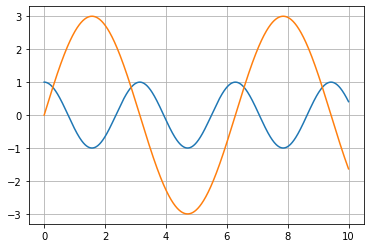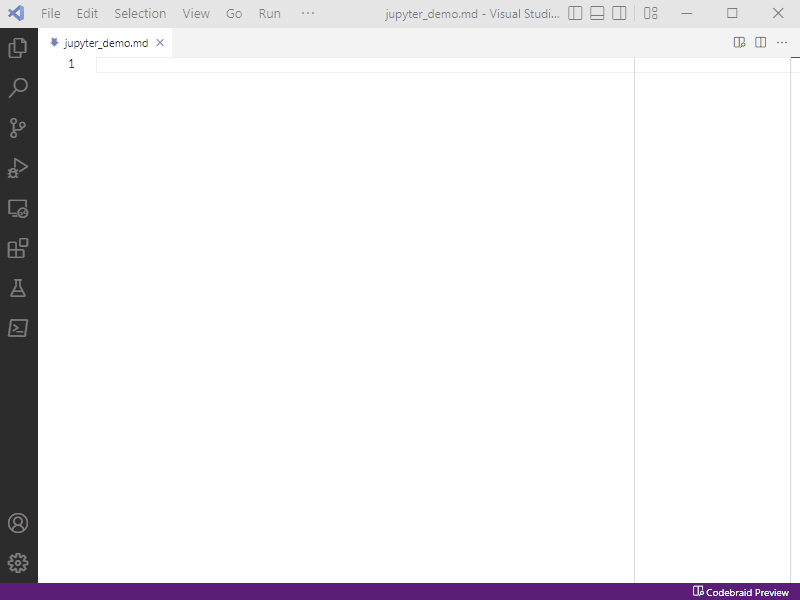# Codebraid – live code in Pandoc Markdown

Create dynamic documents by executing code blocks and inline code in Pandoc Markdown.

• Execute code with Jupyter kernels or with the extensible built-in execution system.
• Display Jupyter rich output like plots and LaTeX math in both block and inline contexts.
• Write pure Pandoc Markdown—no custom syntax or separate preprocessing stage.
• Mix languages freely—use multiple language kernels within the same document and multiple independent sessions per kernel.
• Customize the display of code output—easily modify what is displayed, and display it anywhere in the document using literate programming capabilities.
• Preview documents in VS Code or VSCodium with a dedicated extension supporting scroll sync.
``````---
codebraid:
jupyter: true
---

## Jupyter demo

An example with Matplotlib:

```{.python .cb-nb}
%matplotlib inline
import matplotlib.pyplot as plt
import numpy as np
x = np.linspace(0, 10, 1001)
plt.plot(x, np.cos(2*x))
plt.plot(x, 3*np.sin(x))
plt.grid()
```
``````

## Jupyter demo

An example with Matplotlib:

``````%matplotlib inline
import matplotlib.pyplot as plt
import numpy as np
x = np.linspace(0, 10, 1001)
plt.plot(x, np.cos(2*x))
plt.plot(x, 3*np.sin(x))
plt.grid()``````## Example documents

The documents below demonstrate Codebraid capabilities. For more advanced features, see the end of the Python and Rust examples.

## External resources

The new Codebraid website is currently under development.